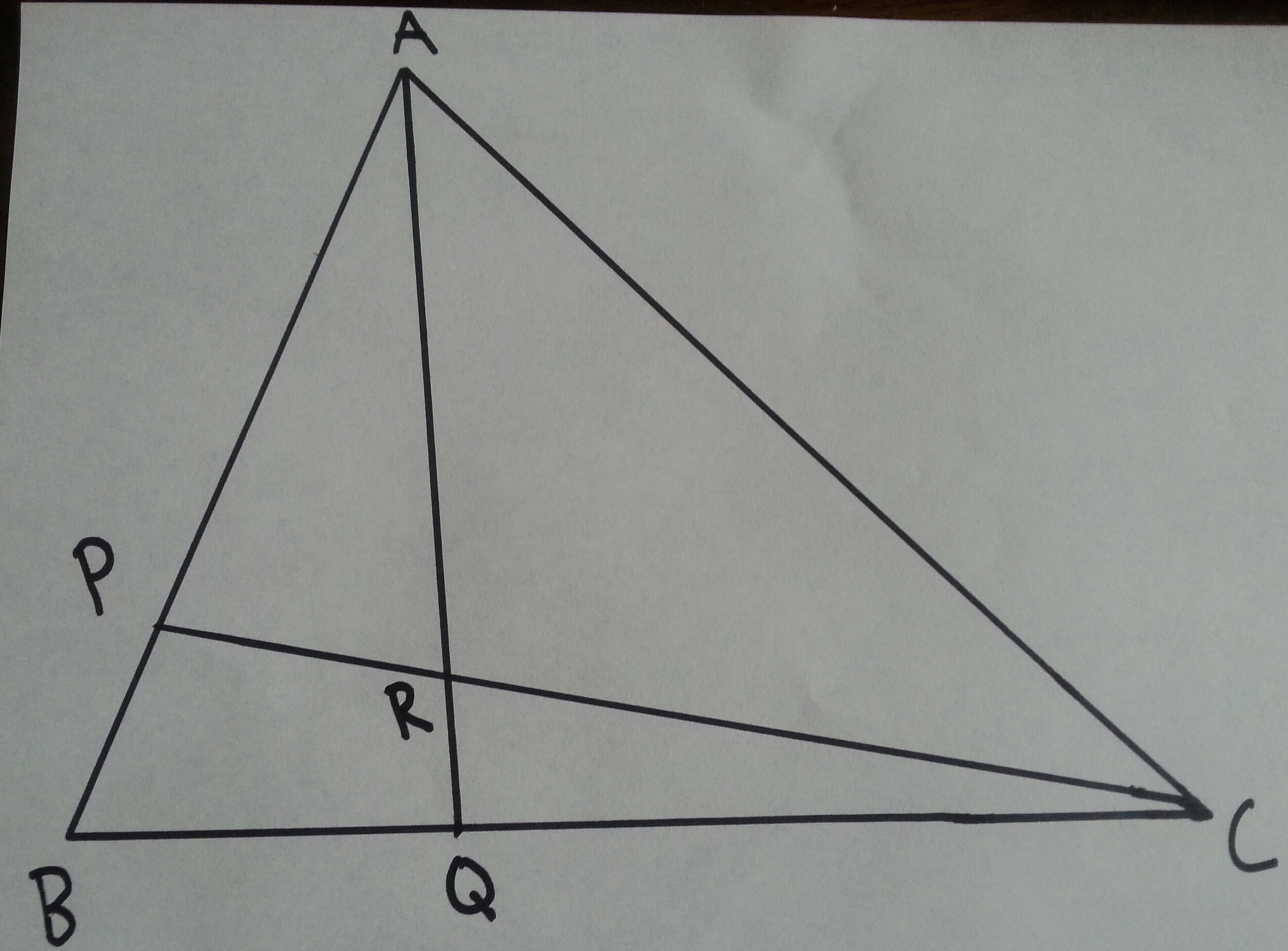# There are no side lengths!

Geometry Level 3Let $ABC$ be a triangle with points $P$ and $Q$ respectively lying on line segments $AB$ and $BC$, such that $\frac{AP}{PB} = 3$ and $\frac{BQ}{QC} = \frac{1}{2}$.

If lines $AQ$ and $CP$ intersect at $R$, the ratio $\frac{AR}{RQ}$ can be expressed as $\frac{a}{b}$ where a and b are coprime integers. Find $a + b$.

Inspiration

×